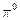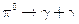Chapter 33, Problem 13PE

Chapter
Section
Textbook Problem

Theis its own antiparticle and decays in the following manner:What is the energy of each (ray if theis at rest when it decays?

To determine

The energy of each γ ray when π0 particle at rest decays by π0γ+γ

Explanation

Given info:

π0 is its own antiparticle and it decays at rest given by π0γ+γ

Calculation:

Initially π0 particle is at rest. So initial momentum of the particle is zero. To conserve momentum

the final momentum of the particle also should be zero. To conserve momentum and energy for the

decay, momenta of the two photons should be equal and opposite. Due to this, the initial energy of

π0 particle is equal to the sum of energies of the two photons

Still sussing out bartleby?

Check out a sample textbook solution.

See a sample solution

The Solution to Your Study Problems

Bartleby provides explanations to thousands of textbook problems written by our experts, many with advanced degrees!

Get Started

Find more solutions based on key concepts